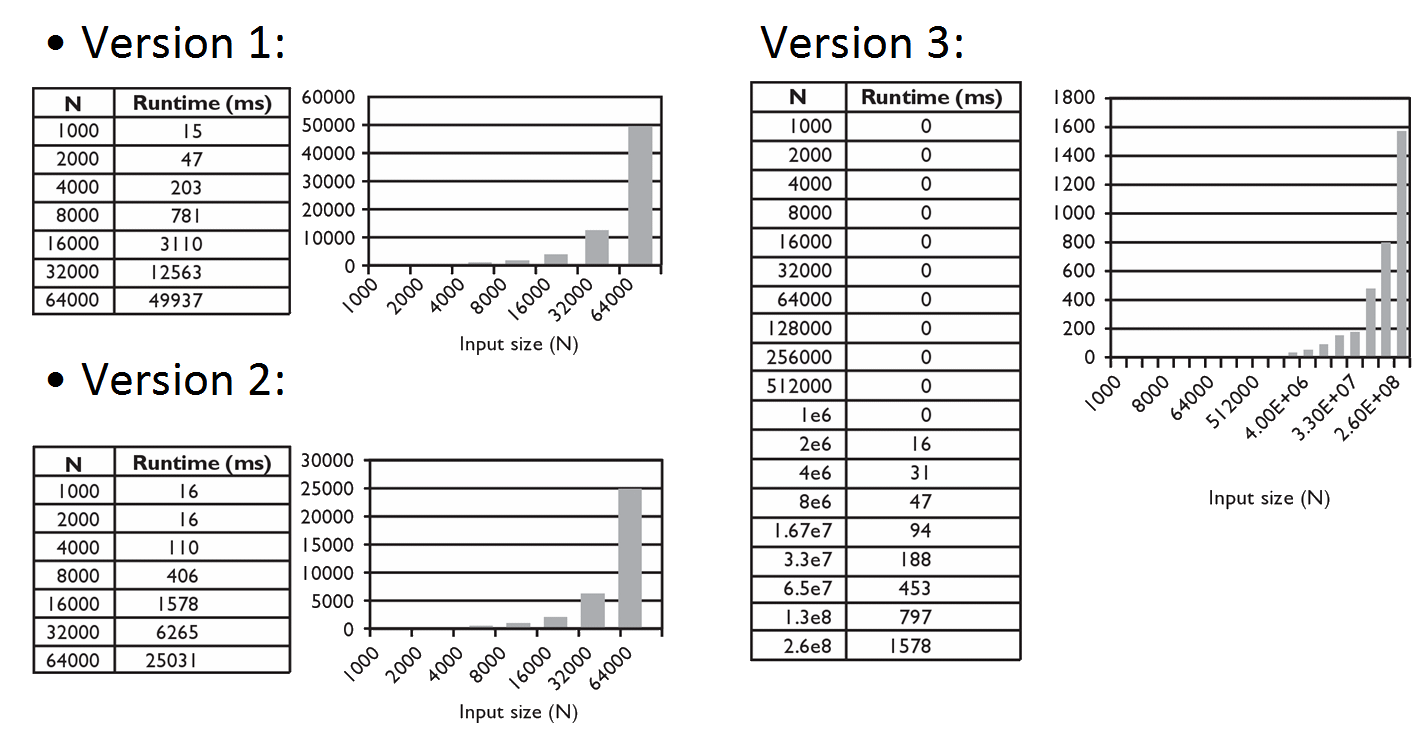### Lecture Preview: Algorithm Analysis and Big-Oh

(Suggested book reading: Programming Abstractions in C++, section 10.2)

Today we will talk about ways to analyze the runtime of algorithms. Many interesting algorithms process data. We are most interested in the algorithm's runtime as the number of elements of data (which we'll call "N") grows. Suppose we measure the runtime of three hypothetical algorithms:Algorithm 3 is obviously much faster, but the main thing to look at is the rate of growth. For algorithms 1 and 2, when you double the input size N, the runtime goes up by a factor of roughly 4. For algorithm 3, when you double N, the runtime goes up by a factor of roughly 2. This growth rate, not the absolute runtime measured on a particular run, is the most important thing to look at when analyzing and choosing algorithms.

We categorize algorithms into groups called complexity classes by looking at their growth rate relative to input size N. Suppose you measure the runtime for a particular N value and then change N.

• If you increase N and the runtime does not change, we say that the algorithm has constant runtime.
• If you increase N by a factor of K and the algorithm's runtime also roughly increases by a factor of K, we say the algorithm has linear growth. (Algorithm 3 above)
• If you increase N by a factor of K and the algorithm's runtime roughly increases by a factor of K*K, we say the algorithm has quadratic growth. (Algorithms 1, 2 above)
• ...

There are many different complexity classes, and as we write and analyze algorithms, we will learn ways of estimating and calculating the complexity class of a given piece of code.

This document and its content are copyright © Marty Stepp, 2017. All rights reserved. Any redistribution, reproduction, transmission, or storage of part or all of the contents in any form is prohibited without the authors' expressed written permission.